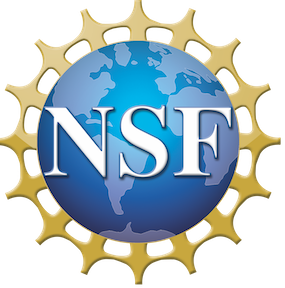# Calculus: Newton, Leibniz, and Robinson Meet Technology# Project DIRACC

## Patrick W. Thompson, Mark Ashbrook

A Work in Progress (Original posting: July, 2015. Latest revision: August, 2019)

Cite as:

Thompson, Patrick W., & Ashbrook, Mark. (2019) Calculus: Newton, Leibniz, and Robinson meet technology. Retrieved from http://patthompson.net/ThompsonCalc/.

## NSF Grant DUE-1625678### Textbook:

Preface for Students

Preface for Instructors

1.0 Installing Graphing Calculator
1.1 Large and Small Quantities
1.2 Large and Small are Relative
1.3 Large Variations are Made of Tiny Variations
1.4 Infinitesimal variations and zooming a graph
1.5 Constant rate of change
2.0 From Trigonometry for High School to Trigonometry for Calculus
2.1 A Graph You've Surely Seen
2.2 The Meaning of x in y = sin(x)
2.3 Positive and Negative Angle Measures
2.4 Angle Measures Greater than 2π or less than -2π
2.5 The Meaning of y in y = sin(x)
2.6 The Tangent Function in Trigonometry
2.7 The Meaning of x and y in y = tan(x)
2.8 Properties of Trigonometric Functions

Chapter 3: Functions, Variables, and Graphs

3.1  Variables, Constants, and Parameters
3.2  Graphing Calculator (GC)
3.3  Using Graphing Calculator
3.4  Typing Mathematical Statements in GC
3.5  What is a Graph?
3.6  How GC Draws Graphs
3.7  Understanding GC's DNA
3.8  (To Be Rewritten)
3.9  Coordinate Systems
3.10  Functions
3.11  Function Notation
3.12  Using Function Notation
3.13  Function Notation and Mathematical Models
3.14  Independent Variable vs. Argument of Function
3.15  Constant Rate of Change & Linear Functions
3.16  Operations on Functions
3.17  Properties of Functions
3.18  Inverse of a Function
3.19  Transformations of Graphs: An Application of Inverse Functions
4.1  Constant Rate of Change
4.2  Differentials as Linear Functions
4.3  Differentials in Action
4.4  Rate of Change at a Moment
4.5  How Small is "Small Enough"?
4.6  Functions Having a Value At Which There Is No Rate of Change at a Moment
4.7  Wrinkly Functions
4.8  Infinitely Wrinkly Functions Having No Rate of Change at Any Moment
4.9  Exact Rate of Change Functions
5.1  Introduction to Accumulation Functions
5.2  Approximate Net Accumulation Functions from Exact Rate of Change Functions
5.3  Exact Net Accumulation Functions from Exact Rate of Change Functions
5.4  Overview of Accumulation from Rate

Chapter 6: Rate of Change from Accumulation

6.1  Approximate Rate of Change in Open Form from Exact Accumulation in Closed Form
6.2  Closed Form Rate of Change from Closed Form Accumulation
6.3  A Garden of Exact Rate of Change Functions
6.4  Big Assumptions That We Made
6.5  The Mean Value Theorem
7.1  Properties of Rate of Change Functions and What They Tell Us
7.2  Higher Order Rate of Change Functions
7.3  Optimization
7.4  Related Rates
7.5  Local Linearity, Indeterminate Forms, and L'Hospital
7.6  More Fundamental Theorem of Calculus -- in Contexts

Chapter 8: More on Integrals

8.0 Review of Terms and Meanings
8.1 The Nature of Integral Problems and Ways to Approach Them
8.2 Regions and Their Signed Areas
8.3 Volumes of Regions in Space
8.4 Arc Length and Surface Area
8.5 Applications of Integrals in the Sciences and Social Sciences

Chapter 9:Integration Techniques

9.0 Motive for Integration Techniques
9.1 Rate of Change Functions, Accumulation Functions, and Antiderivatives
9.2 Undoing the chain rule
9.3 Undoing the product rule
9.4 Antiderivatives of expressions involving trigonometric functions
Chapter 10: Approximating Functions' Values
10.0 Approximating Rate of Change and Accumulation Function's Values
10.1 New Perspectives on Approximating Accumulation Functions from Rate of Change Functions
10.2 Polynomial Approximations, Taylor Series, and Convergence at a Moment
10.2-old Sequences, Series, and Convergence at a Point (traditional)
10.3 Sequences, Series, and Convergence Over an Interval

Chapter 11: Polar Coordinates

11.0 Review of Polar Coordinates
11.1 Graphs in Polar Coordinates and Their Properties
11.2 Coordinate Conversions
11.3 Areas of Regions Bounded by Graphs in Polar Coordinates

Chapter 12: Parametric Relationships and Functions

12.0 From Functions of an Argument to Functions in Relation to Each Other
12.1 Relationships Defined Parametrically
12.2 Calculus of Parametric Relationships

 < Previous Section Home Next Section >